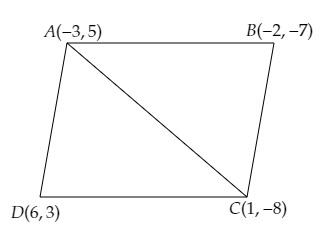# If $A (-3, 5), B (-2, -7), C (1, -8)$ and $D (6, 3)$ are the vertices of a quadrilateral $ABCD$, find its area.

Given:

$A (-3, 5), B (-2, -7), C (1, -8)$ and $D (6, 3)$ are the vertices of a quadrilateral $ABCD$.

To do:

We have to find the area of the quadrilateral.

Solution:

Join $A$ and $C$ to get two triangles $ABC$ and $ADC$.This implies,

Area of quadrilateral $ABCD=$ Area of triangle $ABC+$ Area of triangle $ADC$.

We know that,

Area of a triangle with vertices $(x_1,y_1), (x_2,y_2), (x_3,y_3)$ is given by,

Area of $\Delta=\frac{1}{2}[x_{1}(y_{2}-y_{3})+x_{2}(y_{3}-y_{1})+x_{3}(y_{1}-y_{2})]$

Therefore,

Area of triangle $ABC=\frac{1}{2}[-3(-7+8)+(-2)(-8-5)+1(5+7)]$

$=\frac{1}{2}[-3(1)+(-2)(-13)+1(12)]$

$=\frac{1}{2}[-3+26+12]$

$=\frac{1}{2} \times (35)$

$=\frac{35}{2}$ sq. units.

Area of triangle $ADC=\frac{1}{2}[-3(3+8)+6(-8-5)+1(5-3)]$

$=\frac{1}{2}[-3(11)+6(-13)+1(2)]$

$=\frac{1}{2}[-33-78+2]$

$=\frac{1}{2} \times (-109)$

$=\frac{109}{2}$ sq. units.

Therefore,

The area of the quadrilateral $ABCD=\frac{35}{2}+\frac{109}{2}=\frac{35+109}{2}=72$ sq. units.

The area of the quadrilateral $ABCD$ is $72$ sq. units.

Updated on: 10-Oct-2022

32 Views